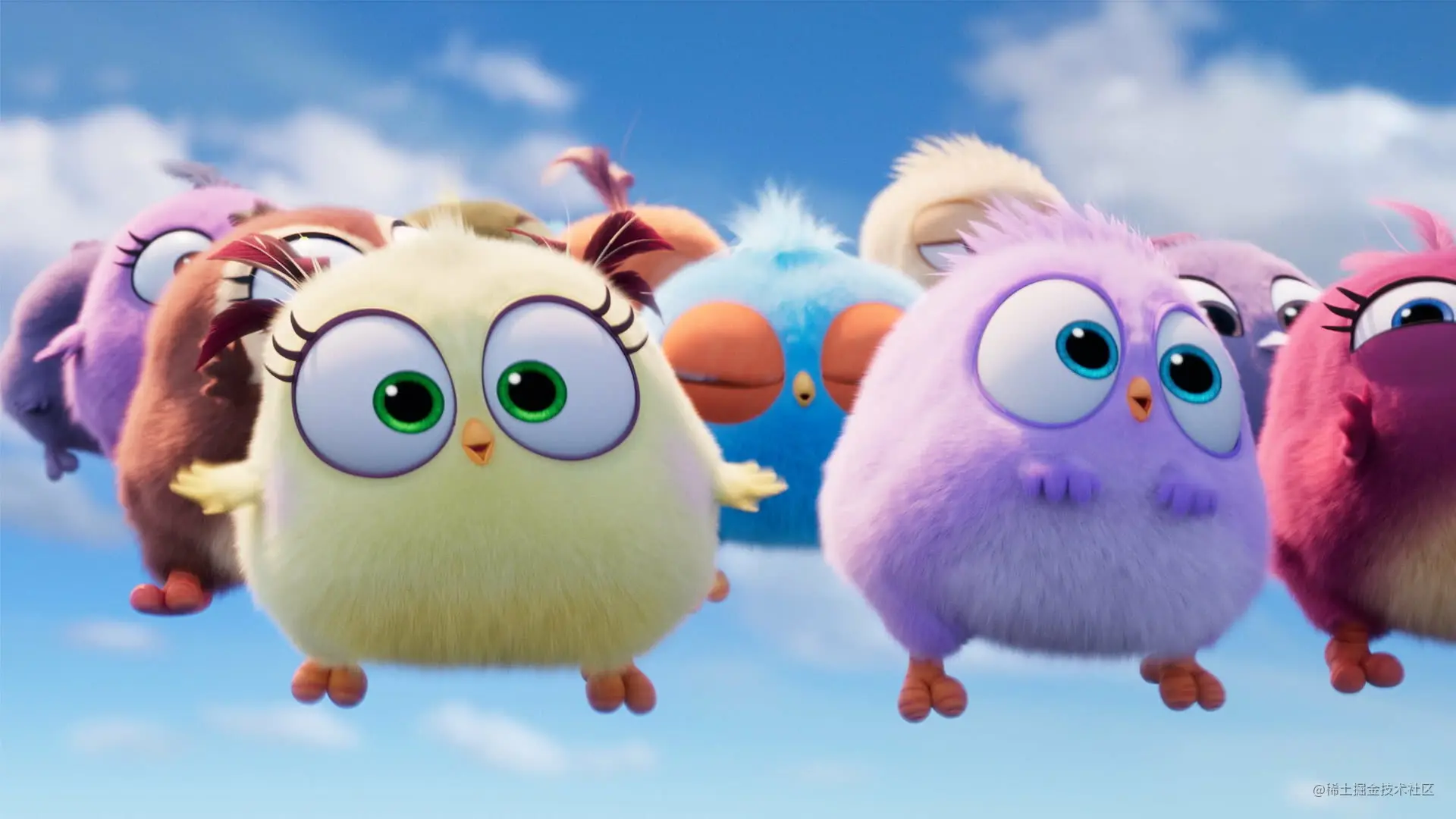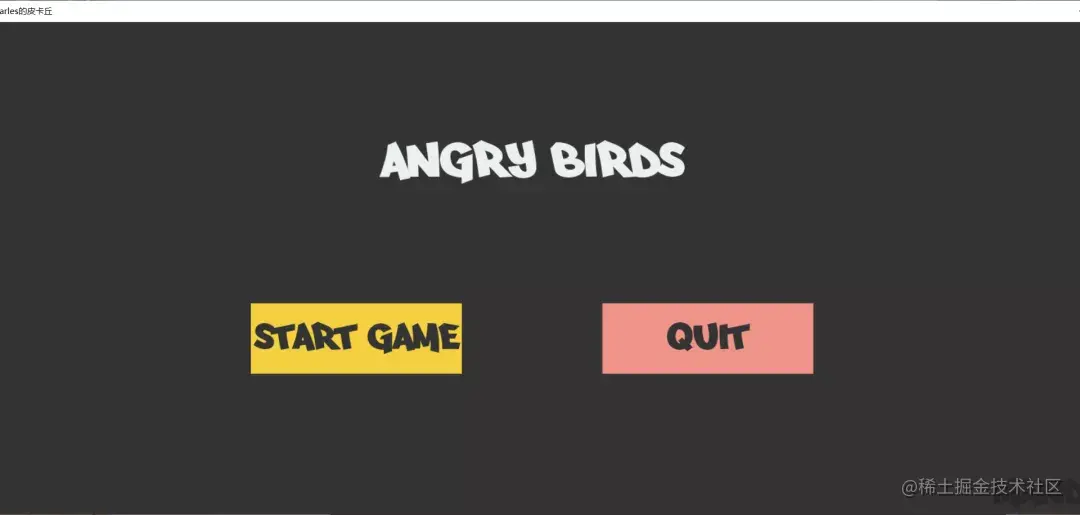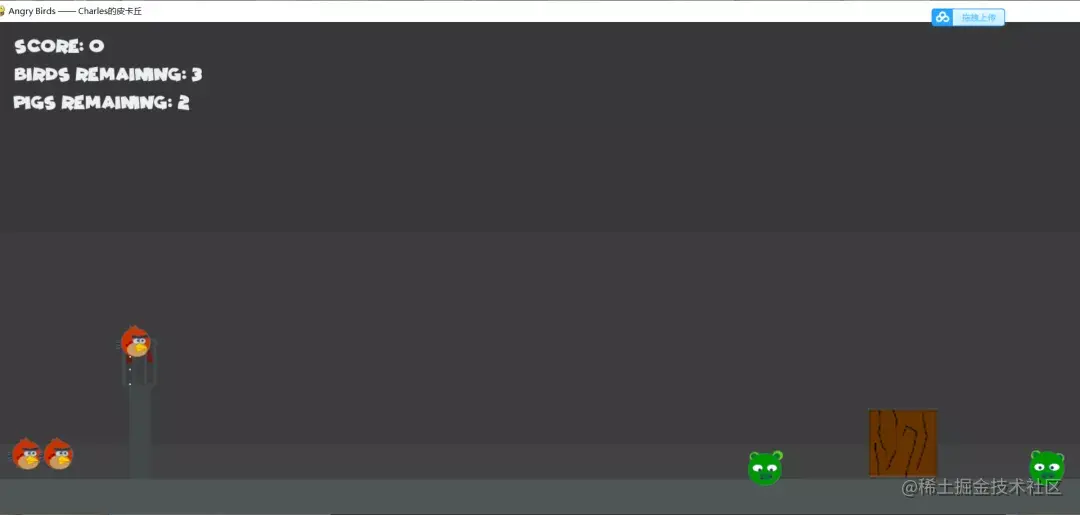# Python game development, pyGame module, python implementation of angry birds

2022-01-30 05:27:57

This article has participated in 「 Digging force Star Program

# Preface

This time I'll take you to write a little angry bird game that was popular for a while .

I don't say much nonsense , Let's start happily ~# development tool

**Python edition ：**3.6.4

Related modules ：

pygame modular ;

As well as some python Built in modules .

# Environment building

install Python And add to environment variable ,pip Install the relevant modules required .

# Introduction of the principle

Here is a brief introduction to the implementation principle of the game . The first is the start interface of the game , It looks like this , It's simple ：There are two main parts , That is, the title of the game and the start and exit buttons of the game , The code implementation of these two parts is as follows ：

``````''' Button class '''
class Button(pygame.sprite.Sprite):
def __init__(self, screen, x, y, width, height, action=None, color_not_active=(189, 195, 199), color_active=(189, 195, 199)):
pygame.sprite.Sprite.__init__(self)
self.x = x
self.y = y
self.width = width
self.height = height
self.action = action
self.screen = screen
self.color_active = color_active
self.color_not_active = color_not_active
''' Add text '''
def addtext(self, text, size=20, font='Times New Roman', color=(0, 0, 0)):
self.font = pygame.font.Font(font, size)
self.text = self.font.render(text, True, color)
self.text_pos = self.text.get_rect()
self.text_pos.center = (self.x + self.width / 2, self.y + self.height / 2)
''' Whether it is selected by the mouse '''
def selected(self):
pos = pygame.mouse.get_pos()
if (self.x < pos < self.x + self.width) and (self.y < pos < self.y + self.height):
return True
return False
''' Draw it on the screen '''
def draw(self):
if self.selected():
pygame.draw.rect(self.screen, self.color_active, (self.x, self.y, self.width, self.height))
else:
pygame.draw.rect(self.screen, self.color_not_active, (self.x, self.y, self.width, self.height))
if hasattr(self, 'text'):
self.screen.blit(self.text, self.text_pos)

''' Text label class '''
class Label(pygame.sprite.Sprite):
def __init__(self, screen, x, y, width, height):
pygame.sprite.Sprite.__init__(self)
self.x = x
self.y = y
self.width = width
self.height = height
self.screen = screen
''' Add text '''
def addtext(self, text, size=20, font='Times New Roman', color=(0, 0, 0)):
self.font = pygame.font.Font(font, size)
self.text = self.font.render(text, True, color)
self.text_pos = self.text.get_rect()
self.text_pos.center = (self.x + self.width / 2, self.y + self.height / 2)
''' Draw it on the screen '''
def draw(self):
if hasattr(self, 'text'):
self.screen.blit(self.text, self.text_pos)
Copy code ``````

In fact, they are relatively simple to implement , A button class is one more button that is selected by the mouse （ That is, when the position of the mouse falls within the area of the button ） Change the color to visually tell the player that the button has been selected .

If the player clicks the exit button （QUIT）, Then quit the game ：

``````def quitgame():
pygame.quit()
sys.exit()
If you click the start game button , Then start the game ：

def startgame():
game_levels = GameLevels(cfg, screen)
game_levels.start()
Copy code ``````

The game interface looks like this ：The way for players to win is to operate a limited number of birds to kill all invading pigs , In other words, use a catapult to launch birds , Let the bird hit all the pigs on the field . If there are still pigs on the field after all the birds are fired, they are not hit , Then the player fails . The code for judging the winning and losing relationship of the game is actually quite simple , It looks something like this ：

``````''' Game status '''
def status(self, pigs, birds):
status_codes = {
'gaming': 0,
'failure': 1,
'victory': 2,
}
if len(pigs) == 0: return status_codes['victory']
elif len(birds) == 0: return status_codes['failure']
else: return status_codes['gaming']
Copy code ``````

next , In order to realize the game , Let's first define all the game sprite classes we need . First , It's our main character , Angry birds ：

``````''' Little bird '''
class Bird(pygame.sprite.Sprite):
def __init__(self, screen, imagepaths, loc_info, velocity=None, color=(255, 255, 255), **kwargs):
pygame.sprite.Sprite.__init__(self)
assert len(loc_info) == 3
assert len(imagepaths) == 1
#  Set the necessary property constants
self.color = color
self.screen = screen
self.loc_info = list(loc_info)
self.imagepaths = imagepaths
self.velocity = VelocityVector() if velocity is None else velocity
self.type = 'bird'
self.fly_path = []
self.elasticity = 0.8
self.is_selected = False
self.inverse_friction = 0.99
self.gravity = VelocityVector(0.2, math.pi)
#  The screen size
self.screen_size = screen.get_rect().size
self.screen_size = (self.screen_size, self.screen_size - 50)
#  Import images
''' Draw it on the screen '''
def draw(self):
for point in self.fly_path:
pygame.draw.ellipse(self.screen, self.color, (point, point, 3, 3), 1)
position = self.loc_info - self.loc_info, self.loc_info - self.loc_info
self.screen.blit(self.image, position)
''' Judge whether it is selected by the mouse '''
def selected(self):
pos = pygame.mouse.get_pos()
dx, dy = pos - self.loc_info, pos - self.loc_info
dist = math.hypot(dy, dx)
if dist < self.loc_info:
return True
return False
''' Load on slingshot '''
self.loc_info, self.loc_info = slingshot.x, slingshot.y
''' Reset position '''
def reposition(self, slingshot):
pos = pygame.mouse.get_pos()
if self.selected:
self.loc_info, self.loc_info = pos, pos
dx, dy = slingshot.x - self.loc_info, slingshot.y - self.loc_info
self.velocity.magnitude = min(int(math.hypot(dx, dy) / 2), 80)
self.velocity.angle = math.pi / 2 + math.atan2(dy, dx)
''' Show the path to launch the bird '''
def projectpath(self):
path = []
bird = Bird(self.screen, self.imagepaths, self.loc_info, velocity=self.velocity)
for i in range(30):
bird.move()
if i % 5 == 0: path.append((bird.loc_info, bird.loc_info))
for point in path:
pygame.draw.ellipse(self.screen, self.color, (point, point, 2, 2))
''' Move the bird '''
def move(self):
#  Change the bird's velocity vector according to gravity
self.velocity = VectorAddition(self.velocity, self.gravity)
self.loc_info += self.velocity.magnitude * math.sin(self.velocity.angle)
self.loc_info -= self.velocity.magnitude * math.cos(self.velocity.angle)
self.velocity.magnitude *= self.inverse_friction
#  The width exceeds the screen
if self.loc_info > self.screen_size - self.loc_info:
self.loc_info = 2 * (self.screen_size - self.loc_info) - self.loc_info
self.velocity.angle *= -1
self.velocity.magnitude *= self.elasticity
elif self.loc_info < self.loc_info:
self.loc_info = 2 * self.loc_info - self.loc_info
self.velocity.angle *= -1
self.velocity.magnitude *= self.elasticity
#  The height exceeds the screen
if self.loc_info > self.screen_size - self.loc_info:
self.loc_info = 2 * (self.screen_size - self.loc_info) - self.loc_info
self.velocity.angle = math.pi - self.velocity.angle
self.velocity.magnitude *= self.elasticity
elif self.loc_info < self.loc_info:
self.loc_info = 2 * self.loc_info - self.loc_info
self.velocity.angle = math.pi - self.velocity.angle
self.velocity.magnitude *= self.elasticity
Copy code ``````

The main consideration to realize it is that birds actually exist in five states :

Queue status , That is, the state of waiting to enter the catapult in the lower left corner , Just stay still ;

Ready state , That is, enter the state where the slingshot is ready to be launched , It needs to keep moving with the mouse , What makes game player know what kind of angle and path it will be when he has adjusted the location of the bird? ;

Flight status , That is, the state after being launched by a slingshot , We need to calculate the bird's flight path and move constantly according to gravity and the bird's initial velocity ;

Collision status , That is, when it hits other objects during flight, such as pigs and wooden piles , The state of motion has changed ;

Stationary state , That is, the state when the bird finally stands still after completing the flight state .

Then let's realize piggy ：

``````''' The pig '''
class Pig(pygame.sprite.Sprite):
def __init__(self, screen, imagepaths, loc_info, velocity=None, **kwargs):
pygame.sprite.Sprite.__init__(self)
assert len(loc_info) == 3
assert len(imagepaths) == 3
#  Set the necessary property constants
self.screen = screen
self.loc_info = list(loc_info)
self.imagepaths = imagepaths
self.velocity = VelocityVector() if velocity is None else velocity
self.type = 'pig'
self.elasticity = 0.8
self.switch_freq = 20
self.animate_count = 0
self.inverse_friction = 0.99
self.gravity = VelocityVector(0.2, math.pi)
#  The screen size
self.screen_size = screen.get_rect().size
self.screen_size = (self.screen_size, self.screen_size - 50)
#  Import images
self.pig_images = []
for imagepath in imagepaths: self.pig_images.append(pygame.image.load(imagepath))
#  Set the current image
self.image = random.choice(self.pig_images[:2])
''' Draw it on the screen '''
def draw(self):
self.animate_count += 1
if (self.animate_count % self.switch_freq == 0) and (not self.is_dead):
self.animate_count = 0
self.image = random.choice(self.pig_images[:2])
position = self.loc_info - self.loc_info, self.loc_info - self.loc_info
self.screen.blit(self.image, position)
''' Mobile pig '''
def move(self):
#  Change the pig's velocity vector according to gravity
self.velocity = VectorAddition(self.velocity, self.gravity)
self.loc_info += self.velocity.magnitude * math.sin(self.velocity.angle)
self.loc_info -= self.velocity.magnitude * math.cos(self.velocity.angle)
self.velocity.magnitude *= self.inverse_friction
#  The width exceeds the screen
if self.loc_info > self.screen_size - self.loc_info:
self.loc_info = 2 * (self.screen_size - self.loc_info) - self.loc_info
self.velocity.angle *= -1
self.velocity.magnitude *= self.elasticity
elif self.loc_info < self.loc_info:
self.loc_info = 2 * self.loc_info - self.loc_info
self.velocity.angle *= -1
self.velocity.magnitude *= self.elasticity
#  The height exceeds the screen
if self.loc_info > self.screen_size - self.loc_info:
self.loc_info = 2 * (self.screen_size - self.loc_info) - self.loc_info
self.velocity.angle = math.pi - self.velocity.angle
self.velocity.magnitude *= self.elasticity
elif self.loc_info < self.loc_info:
self.loc_info = 2 * self.loc_info - self.loc_info
self.velocity.angle = math.pi - self.velocity.angle
self.velocity.magnitude *= self.elasticity
''' The pig died '''
self.image = self.pig_images[-1]
Copy code ``````

Pigs mainly include three states in the game ：

Stationary state , That is, the state of being stationary somewhere when you are not hit ;

The state of motion after being hit , That is, after being hit by other objects, it also runs together according to the principle of momentum conservation ;

The static state after being hit , That is, the state in which motion is caused by being hit and then returns to rest 、

The implementation principle of wood blocks in the game is similar to that of pigs ：

``````''' The wood in the map '''
class Block(pygame.sprite.Sprite):
def __init__(self, screen, imagepaths, loc_info, velocity=None, **kwargs):
pygame.sprite.Sprite.__init__(self)
assert len(loc_info) == 3
assert len(imagepaths) == 2
#  Set the necessary property constants
self.type = 'block'
self.screen = screen
self.loc_info = list(loc_info)
self.imagepaths = imagepaths
self.velocity = VelocityVector() if velocity is None else velocity
self.elasticity = 0.7
self.is_destroyed = False
self.inverse_friction = 0.99
self.gravity = VelocityVector(0.2, math.pi)
#  Import images
self.block_images = []
for imagepath in imagepaths: self.block_images.append(pygame.transform.scale(pygame.image.load(imagepath), (100, 100)))
#  The screen size
self.screen_size = screen.get_rect().size
self.screen_size = (self.screen_size, self.screen_size - 50)
#  Set the current image
self.image = self.block_images
self.rect = self.image.get_rect()
''' Draw it on the screen '''
def draw(self):
pygame.transform.rotate(self.image, self.rotate_angle)
self.screen.blit(self.image, (self.loc_info - self.rect.width // 2, self.loc_info))
''' Set to damaged state '''
def setdestroy(self):
self.is_destroyed = True
self.image = self.block_images
''' Move the wooden block '''
def move(self):
#  Change the velocity vector of the wood block according to gravity
self.velocity = VectorAddition(self.velocity, self.gravity)
self.loc_info += self.velocity.magnitude * math.sin(self.velocity.angle)
self.loc_info -= self.velocity.magnitude * math.cos(self.velocity.angle)
self.velocity.magnitude *= self.inverse_friction
#  The width exceeds the screen
if self.loc_info > self.screen_size - self.rect.width:
self.loc_info = 2 * (self.screen_size - self.rect.width) - self.loc_info
self.velocity.angle *= -1
self.rotate_angle = -self.velocity.angle
self.velocity.magnitude *= self.elasticity
elif self.loc_info < self.rect.width:
self.loc_info = 2 * self.rect.width - self.loc_info
self.velocity.angle *= -1
self.rotate_angle = -self.velocity.angle
self.velocity.magnitude *= self.elasticity
#  The height exceeds the screen
if self.loc_info > self.screen_size - self.rect.height:
self.loc_info = 2 * (self.screen_size - self.rect.height) - self.loc_info
self.velocity.angle = math.pi - self.velocity.angle
self.rotate_angle = math.pi - self.velocity.angle
self.velocity.magnitude *= self.elasticity
elif self.loc_info < self.rect.height:
self.loc_info = 2 * self.rect.height - self.loc_info
self.velocity.angle = math.pi - self.velocity.angle
self.rotate_angle = math.pi - self.velocity.angle
self.velocity.magnitude *= self.elasticity
Copy code ``````

Last , Let's just implement the wall and slingshot ：

``````''' Slingshot '''
class Slingshot(pygame.sprite.Sprite):
def __init__(self, screen, x, y, width, height, color=(66, 73, 73), line_color=(100, 30, 22), **kwargs):
pygame.sprite.Sprite.__init__(self)
self.x = x
self.y = y
self.color = color
self.width = width
self.height = height
self.screen = screen
self.line_color = line_color
self.type = 'slingshot'
''' Draw it on the screen '''
def draw(self, bird=None):
pygame.draw.rect(self.screen, self.color, (self.x, self.y + self.height * 1 / 3, self.width, self.height * 2 / 3))
if bird is not None and bird.is_loaded:
pygame.draw.line(self.screen, self.line_color, (self.x, self.y + self.height / 6), (bird.loc_info, bird.loc_info + bird.loc_info / 2), 10)
pygame.draw.line(self.screen, self.line_color, (self.x + self.width, self.y + self.height / 6), (bird.loc_info + bird.loc_info, bird.loc_info + bird.loc_info / 2), 10)
pygame.draw.rect(self.screen, self.color, (self.x - self.width / 4, self.y, self.width / 2, self.height / 3), 5)
pygame.draw.rect(self.screen, self.color, (self.x + self.width * 3 / 4, self.y, self.width / 2, self.height / 3), 5)

''' wall '''
class Slab(pygame.sprite.Sprite):
def __init__(self, screen, imagepaths, x, y, width, height, color=(255, 255, 255)):
pygame.sprite.Sprite.__init__(self)
self.x = x
self.y = y
self.color = color
self.width = width
self.height = height
self.screen = screen
self.imagepaths = imagepaths
if self.width > self.height:
else:
self.image = pygame.transform.scale(self.image, (self.width, self.height))
self.type = 'wall'
''' Draw it on the screen '''
def draw(self):
self.screen.blit(self.image, (self.x, self.y))
Copy code ``````

thus , We have completed the definition of all game elves , You can start to realize the main cycle of the game , The specific code implementation is as follows ：

``````''' Start the game '''
def start(self):
#  Import all game sprites
birds, pigs, blocks, walls = game_sprites['birds'], game_sprites['pigs'], game_sprites['blocks'], game_sprites['walls']
slingshot = Slingshot(self.screen, 200, self.screen_size - 200, 30, 200)
score_label = Label(self.screen, 50, 10, 100, 50)
score_label.addtext(f'SCORE: {self.score}', 25, self.cfg.FONTPATH['Comic_Kings'], (236, 240, 241))
birds_remaining_label = Label(self.screen, 120, 50, 100, 50)
birds_remaining_label.addtext(f"BIRDS REMAINING: {len(birds)}", 25, self.cfg.FONTPATH['Comic_Kings'], (236, 240, 241))
pigs_remaining_label = Label(self.screen, 110, 90, 100, 50)
pigs_remaining_label.addtext(f"PIGS REMAINING: {len(pigs)}", 25, self.cfg.FONTPATH['Comic_Kings'], (236, 240, 241))
carles_label = Label(self.screen, self.screen_size - 270, self.screen_size - 20, 300, 100)
carles_label.addtext('CARLES', 60, self.cfg.FONTPATH['arfmoochikncheez'], (113, 125, 126))
#  The main cycle of the game
clock = pygame.time.Clock()
blocks_to_remove, pigs_to_remove = [], []
while True:
# -- Key detection
for event in pygame.event.get():
if event.type == pygame.QUIT:
self.quitgame()
elif event.type == pygame.KEYDOWN:
if event.key == pygame.K_q:
self.quitgame()
elif event.key == pygame.K_r:
self.start()
elif event.key == pygame.K_p or event.key == pygame.K_ESCAPE:
self.pauseinterface()
elif event.type == pygame.MOUSEBUTTONDOWN:
if birds.selected():
birds.is_selected = True
elif event.type == pygame.MOUSEBUTTONUP:
if birds.is_selected:
birds.is_selected = False
birds.start_flying = True
# -- Background color fill
color = self.cfg.BACKGROUND_COLOR
for i in range(3):
color = (color + 5, color + 5, color + 5)
pygame.draw.rect(self.screen, color, (0, i * 300, self.screen_size, 300))
pygame.draw.rect(self.screen, (77, 86, 86), (0, self.screen_size, self.screen_size, 50))
# -- Determine if the game is over , If not, import a new bird
if (not birds.is_loaded) and self.still(pigs + birds + blocks):
birds.pop(0)
if self.status(pigs, birds) == 2:
self.score += len(birds) * 100
self.switchlevelinterface()
elif self.status(pigs, birds) == 1:
self.failureinterface()
birds.start_flying = False
# -- Reset the bird's position
if birds.is_selected:
birds.reposition(slingshot)
if hasattr(birds, 'start_flying') and birds.start_flying:
# -- Slingshot
slingshot.draw(birds)
# -- Judge whether the pig hit a stake
for i in range(len(pigs)):
for j in range(len(blocks)):
pig_magnitude_1, block_magnitude_1 = pigs[i].velocity.magnitude, blocks[j].velocity.magnitude
pigs[i], blocks[j], is_collision = self.collision(pigs[i], blocks[j])
pig_magnitude_2, block_magnitude_2 = pigs[i].velocity.magnitude, blocks[j].velocity.magnitude
if is_collision:
if abs(pig_magnitude_2 - pig_magnitude_2) > 2:
blocks_to_remove.append(blocks[j])
blocks[j].setdestroy()
if abs(block_magnitude_2 - block_magnitude_1) > 2:
pigs_to_remove.append(pigs[i])
# -- Judge whether the bird hit the stake
for i in range(len(birds)):
if not (birds[i].is_loaded or birds[i].velocity.magnitude == 0):
for j in range(len(blocks)):
bird_magnitude_1, block_magnitude_1 = birds[i].velocity.magnitude, blocks[j].velocity.magnitude
birds[i], blocks[j], is_collision = self.collision(birds[i], blocks[j])
bird_magnitude_2, block_magnitude_2 = birds[i].velocity.magnitude, blocks[j].velocity.magnitude
if is_collision:
if abs(bird_magnitude_1 - bird_magnitude_2) > 2:
if blocks[j] not in blocks_to_remove:
blocks_to_remove.append(blocks[j])
blocks[j].setdestroy()
# -- Judge whether the pig hit the pig or the pig hit the wall
for i in range(len(pigs)):
pigs[i].move()
for j in range(i+1, len(pigs)):
pig1_magnitude_1, pig2_magnitude_1 = pigs[i].velocity.magnitude, pigs[j].velocity.magnitude
pigs[i], pigs[j], is_collision = self.collision(pigs[i], pigs[j])
pig1_magnitude_2, pig2_magnitude_2 = pigs[i].velocity.magnitude, pigs[j].velocity.magnitude
if abs(pig1_magnitude_1 - pig1_magnitude_2) > 2:
if pigs[j] not in pigs_to_remove:
pigs_to_remove.append(pigs[j])
if abs(pig2_magnitude_1 - pig2_magnitude_2) > 2:
if pigs[i] not in pigs_to_remove:
pigs_to_remove.append(pigs[i])
for wall in walls: pigs[i] = self.collision(pigs[i], wall)
pigs[i].draw()
# -- Judge whether the bird hit the pig or the bird hit the wall
for i in range(len(birds)):
if (not birds[i].is_loaded) and (birds[i].velocity.magnitude):
birds[i].move()
for j in range(len(pigs)):
bird_magnitude_1, pig_magnitude_1 = birds[i].velocity.magnitude, pigs[j].velocity.magnitude
birds[i], pigs[j], is_collision = self.collision(birds[i], pigs[j])
bird_magnitude_2, pig_magnitude_2 = birds[i].velocity.magnitude, pigs[j].velocity.magnitude
if is_collision:
if abs(bird_magnitude_2 - bird_magnitude_1) > 2:
if pigs[j] not in pigs_to_remove:
pigs_to_remove.append(pigs[j])
for wall in walls: birds[i] = self.collision(birds[i], wall)
birds[i].draw()
# -- Judge whether the stake hit the stake or the stake hit the wall
for i in range(len(blocks)):
for j in range(i+1, len(blocks)):
block1_magnitude_1, block2_magnitude_1 = blocks[i].velocity.magnitude, blocks[j].velocity.magnitude
blocks[i], blocks[j], is_collision = self.collision(blocks[i], blocks[j])
block1_magnitude_2, block2_magnitude_2 = blocks[i].velocity.magnitude, blocks[j].velocity.magnitude
if is_collision:
if abs(block1_magnitude_2 - block1_magnitude_1) > 2:
if blocks[j] not in blocks_to_remove:
blocks_to_remove.append(blocks[j])
blocks[j].setdestroy()
if abs(block2_magnitude_2 - block2_magnitude_1) > 2:
if blocks[i] not in blocks_to_remove:
blocks_to_remove.append(blocks[i])
blocks[i].setdestroy()
blocks[i].move()
for wall in walls: blocks[i] = self.collision(blocks[i], wall)
blocks[i].draw()
# -- wall
for wall in walls: wall.draw()
# -- According to the text
score_label.addtext(f'SCORE: {self.score}', 25, self.cfg.FONTPATH['Comic_Kings'], (236, 240, 241))
score_label.draw()
birds_remaining_label.addtext(f"BIRDS REMAINING: {len(birds)}", 25, self.cfg.FONTPATH['Comic_Kings'], (236, 240, 241))
birds_remaining_label.draw()
pigs_remaining_label.addtext(f"PIGS REMAINING: {len(pigs)}", 25, self.cfg.FONTPATH['Comic_Kings'], (236, 240, 241))
pigs_remaining_label.draw()
carles_label.draw()
# -- Screen refresh
pygame.display.update()
clock.tick(self.cfg.FPS)
# -- Delete invalid element
if self.still(birds + pigs + blocks):
for pig in pigs_to_remove:
if pig in pigs:
pigs.remove(pig)
self.score += 100
for block in blocks_to_remove:
if block in blocks:
blocks.remove(block)
self.score += 50
pigs_to_remove = []
blocks_to_remove = []
Copy code ``````

Key detection, collision detection and real-time update of game status such as some scores

This is the end of the article , Thanks for watching ,Python27 A series of small games , Share the next article Python Memory flop games

To thank readers , I want to share with you some of my recent collections of programming dry goods , Give back to every reader , I hope I can help you .

For the complete source code, see the relevant documents of the introduction to the home page ~

# Look back in the past

Python Realize the Bomber game

Python Realize the classic bean eating games

Python Real dinosaur jump games now

Python Realize the simple version of aircraft war games

Python Realize Tetris games

Python Alien invasion games

Python Realization “ Bunny and Bun” game

Python Achieve the classic 90 Tanks war

Python Achieve tower defense games